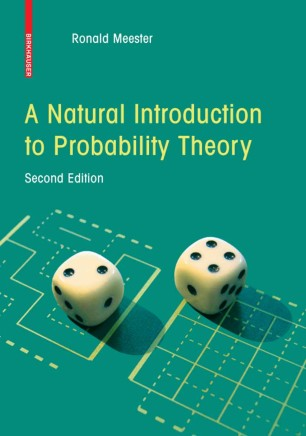# A Natural Introduction to Probability Theory

• Ronald MeesterTextbook

1. Front Matter
Pages i-x
2. Pages 1-33
3. Pages 35-70
4. Pages 71-79
5. Pages 81-88
6. Pages 89-92
7. Pages 93-135
8. Pages 137-151
9. Pages 153-166
10. Pages 167-182
11. Pages 183-186
12. Back Matter
Pages 187-197

### Introduction

According to Leo Breiman (1968), probability theory has a right and a left hand. The right hand refers to rigorous mathematics, and the left hand refers to ‘pro- bilistic thinking’. The combination of these two aspects makes probability theory one of the most exciting ?elds in mathematics. One can study probability as a purely mathematical enterprise, but even when you do that, all the concepts that arisedo haveameaningontheintuitivelevel.Forinstance,wehaveto de?newhat we mean exactly by independent events as a mathematical concept, but clearly, we all know that when we ?ip a coin twice, the event that the ?rst gives heads is independent of the event that the second gives tails. Why have I written this book? I have been teaching probability for more than ?fteen years now, and decided to do something with this experience. There are already many introductory texts about probability, and there had better be a good reason to write a new one. I will try to explain my reasons now.

### Keywords

Excel Limit theorems Probability theory Random variable Random walk coding mathematical statistics measure theory

#### Authors and affiliations

• Ronald Meester
• 1
1. 1.Faculteit der Exacte WetenschappenVrije UniversiteitAmsterdamThe Netherlands

Industry Sectors
Pharma
Biotechnology